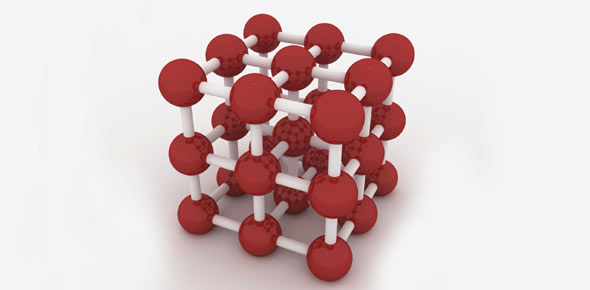# Atoms, The Nucleus And The Mole

9 Questions | Attempts: 76SettingsChemistry students, assemble! Today we’ll be having a look at a number of different topics under the overall study of nuclear chemistry, including atoms, the nucleus and the mole. Do you have a firm enough grip on each of these topics? Take this quiz and find out!

• 1.
Which of the following has an odd number of both protons and neutrons?
• A.

Helium-4

• B.

Lithium-7

• C.

Boron-10

• D.

Carbon-13

• 2.
If you have 5g each of Li, Na, K, and Rb, for which element will you have the largest number of moles?
• A.

Lithium

• B.

Sodium

• C.

Potassium

• D.

Rubidium

• 3.
If you have three times the mass of carbon as you have helium, which of the following is true?
• A.

You have nearly equal numbers of carbon atoms as helium atoms.

• B.

You have more atoms of carbon.

• C.

You have more atoms of helium.

• D.

You cannot know a relationship between carbon atoms and helium atoms.

• 4.
In positron emission, what is the resulting change in the nucleus?
• A.

Atomic number increases by 1.

• B.

Atomic number decreases by 1.

• C.

Atomic number decreases by 2.

• D.

Atomic number stays the same.

• 5.
Who discovered the charge to mass ratio for electrons?
• A.

J.J. Thomson

• B.

Ernest Rutherford

• C.

John Dalton

• D.

Robert Millikan

• 6.
Which of the following pairs of compounds could be used to explain the law of multiple proportions?
• A.

H2O and H2O2

• B.

CH4 and CO2

• C.

NaCl and KCl

• D.

SO3 and H2S

• 7.
What experiment resulted to the discovery of the nucleus?
• A.

Gold foil (or alpha scattering) experiment

• B.

Cathode ray tubes

• C.

Oil drop experiment

• D.

Mass spectroscopy

• 8.
How many electrons are in a magnesium-24 with a charge of 2+?
• A.

10

• B.

12

• C.

14

• D.

22

• 9.
How are ions formed?
• A.

By gaining or losing electrons

• B.

By gaining or losing protons

• C.

By beta decay

• D.

By positron emission

## Related TopicsBack to top
×

Wait!
Here's an interesting quiz for you.